# Challenge Task 4 At what pivot point O should a uniform rod be suspended so that the frequency of its oscillations as a physical pendulum is the maximum? What is this frequency? mg Challenge Tas...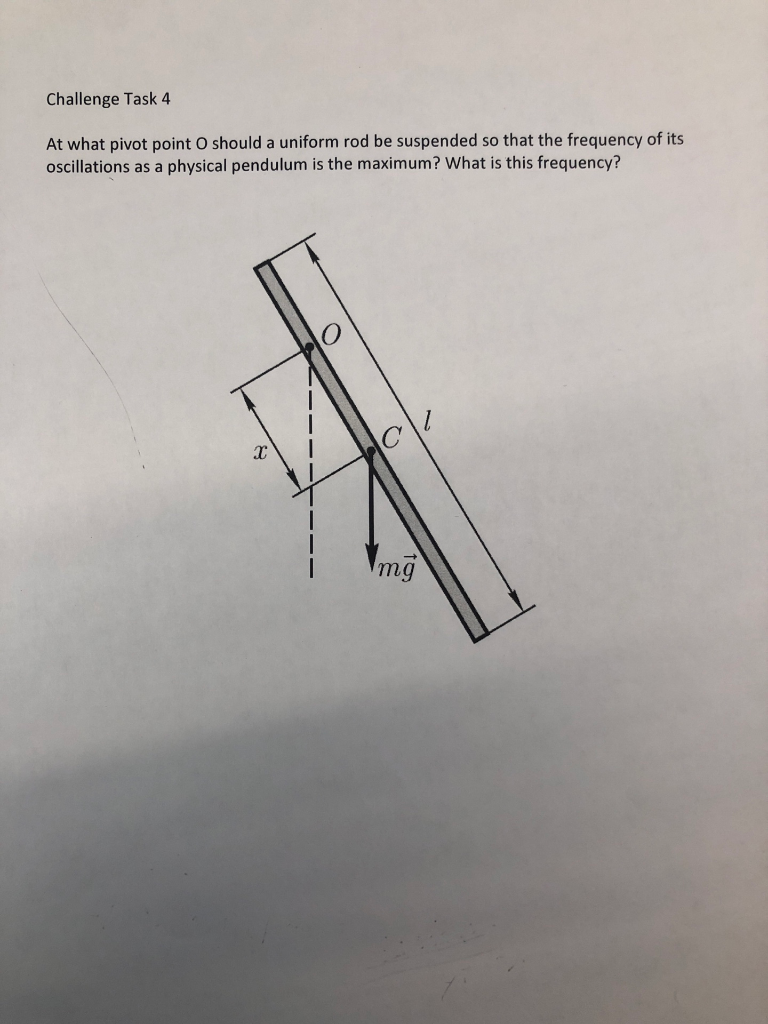Challenge Task 4 At what pivot point O should a uniform rod be suspended so that the frequency of its oscillations as a physical pendulum is the maximum? What is this frequency? mg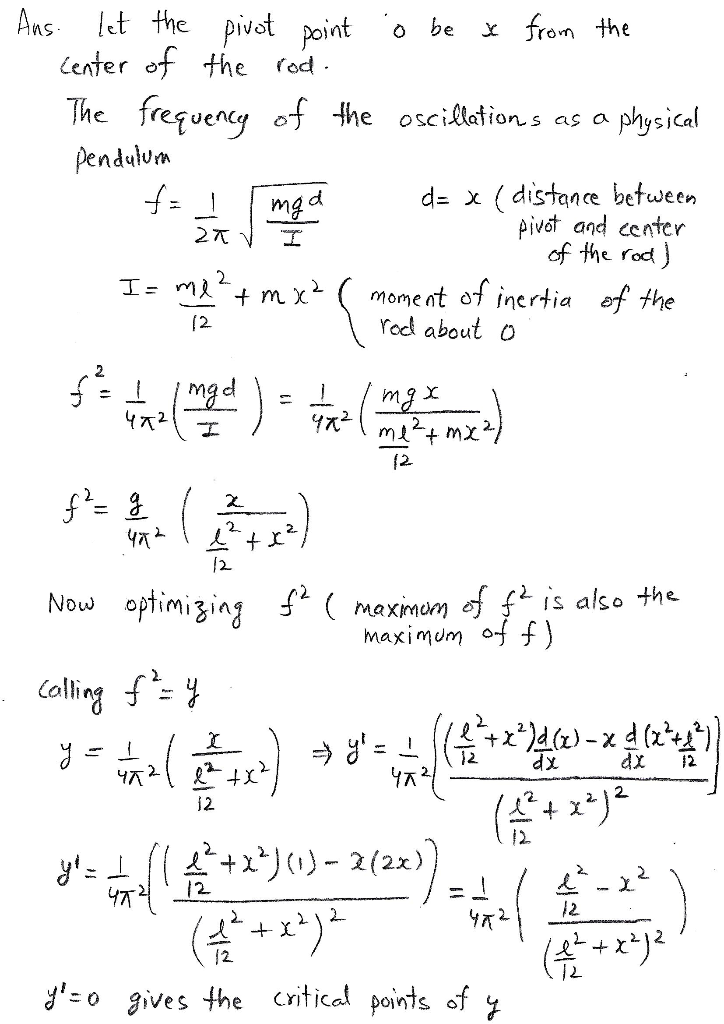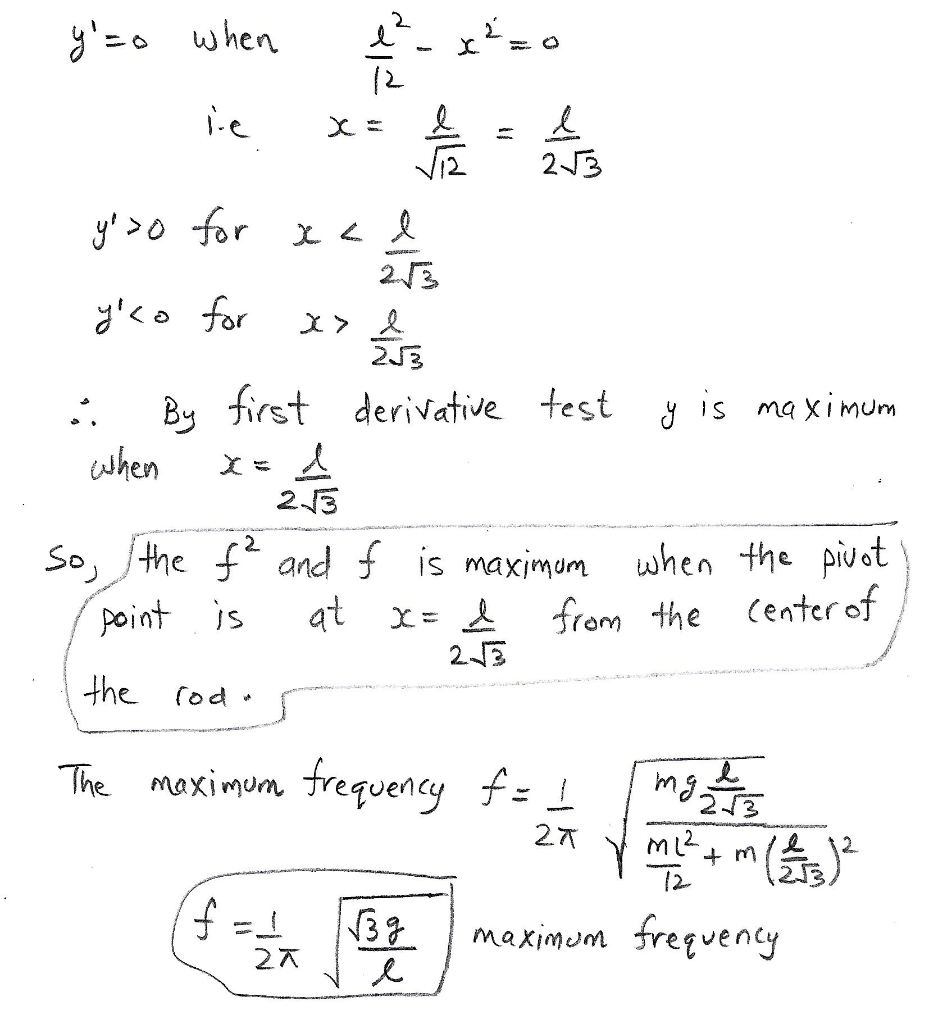##### Add Answer of: Challenge Task 4 At what pivot point O should a uniform rod be suspended so that the frequency of its oscillations as a physical pendulum is the maximum? What is this frequency? mg Challenge Tas...
Similar Homework Help Questions
• ### Q4 A ring of mass M and radius R is attached so that it can pivot asone of a rod of mass M and length 2R. the syste...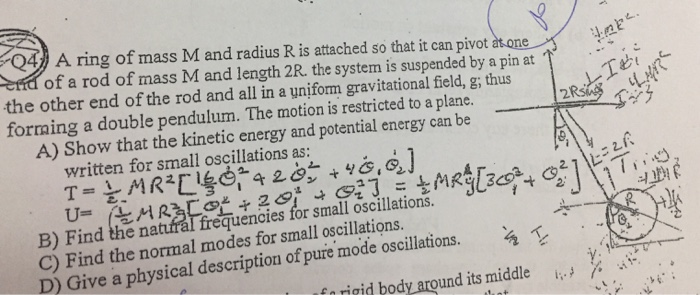Q4 A ring of mass M and radius R is attached so that it can pivot asone of a rod of mass M and length 2R. the system is suspended by a pin at the other end of the rod and all in a uniform gravitational field,g; thus forming a double pendulum. The motion is restricted to a plane A) Show that the kinetic energy and potential energy can be written for small oscillations as: B) Find the natural frequencies...

• ### A compound pendulum is assembled as follows A mass of 2 kg, having moment oj nerta of O, 1 kg. ma...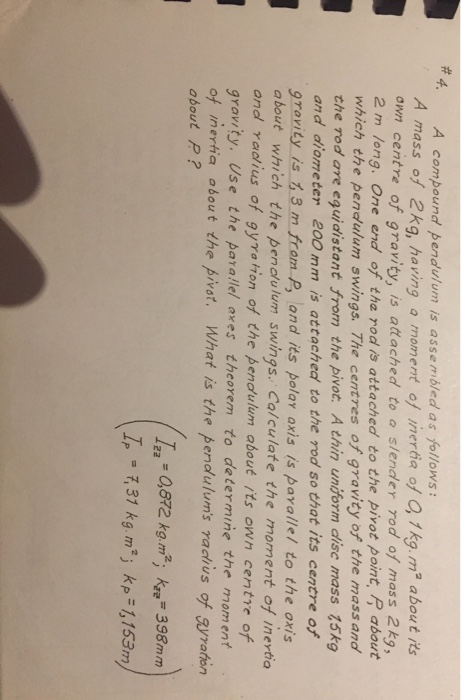A compound pendulum is assembled as follows A mass of 2 kg, having moment oj nerta of O, 1 kg. ma about its own centre of gravity, is attached to o slender rod of mass 2k, 2 m long. One end of tha rod is attached to the pivot point,R about which the pendulum swings. The centres of gravity of the mass and the rod are eguidistant from the pivot. A thin uniform disc mass 15kg and diometer 200 mm...

• ### A uniform circular disk whose radius R is 32.0 cm is suspended as a physical pendulum from a point on its rim

A uniform circular disk whose radius R is 32.0 cm is suspended as a physical pendulum from a point on its rim. (a) What is its period of oscillation? __ s (b) At what radial distance r < R is there a point of suspension that gives the same period? __ cm in the book.. it gives me a hint: ..... the period of oscillation is given by T = 2pi sqrt(I/mgh), where I is the rotational inertia of the...

• ### L- Pivot Point 13.) A uniform, thin rod of length L and mass M is allowed to pivot about its end,...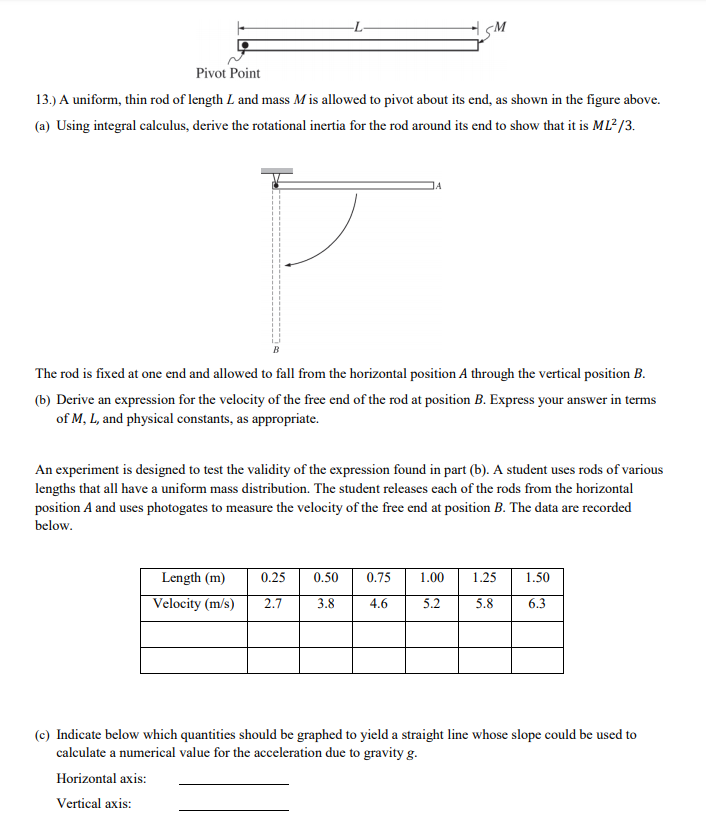L- Pivot Point 13.) A uniform, thin rod of length L and mass M is allowed to pivot about its end, as shown in the figure above (a) Using integral calculus, derive the rotational inertia for the rod around its end to show that it is ML2/3 The rod is fixed at one end and allowed to fall from the horizontal position A through the vertical position B. (b) Derive an expression for the velocity of the free end of...

Need Online Homework Help?